StatLect

# Generalized least squares

The generalized least squares (GLS) estimator of the coefficients of a linear regression is a generalization of the ordinary least squares (OLS) estimator. It is used to deal with situations in which the OLS estimator is not BLUE (best linear unbiased estimator) because one of the main assumptions of the Gauss-Markov theorem, namely that of homoskedasticity and absence of serial correlation, is violated. In such situations, provided that the other assumptions of the Gauss-Markov theorem are satisfied, the GLS estimator is BLUE.## Setting

The linear regression iswhere:

•is anvector of outputs (is the sample size);

•is anmatrix of regressors (is the number of regressors);

•is thevector of regression coefficients to be estimated;

•is anvector of error terms.

We assume that:

1.has full rank;

2.;

3., whereis asymmetric positive definite matrix.

These assumptions are the same made in the Gauss-Markov theorem in order to prove that OLS is BLUE, except for assumption 3.

In the Gauss-Markov theorem, we make the more restrictive assumption thatwhereis theidentity matrix. The latter assumption means that the errors of the regression are homoskedastic (they all have the same variance) and uncorrelated (their covariances are all equal to zero).

Instead, we now allow for heteroskedasticity (the errors can have different variances) and correlation (the covariances between errors can be different from zero).

## The GLS estimator

Sinceis symmetric and positive definite, there is an invertible matrixsuch thatIf we pre-multiply the regression equation by, we obtainDefineso that the transformed regression equation can be written asThe following proposition holds.

Proposition The OLS estimator of the coefficients of the transformed regression equation, called generalized least squares estimator, is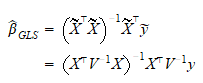Furthermore,is BLUE (best linear unbiased).

Proof

The estimator is derived from the formula of the OLS estimator of the coefficients of the transformed regression equation: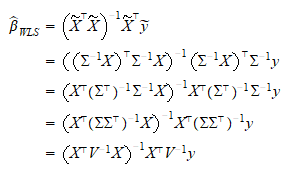Furthermore, we have thatis full-rank (becauseandare). Moreover,and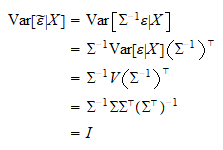Therefore, the transformed regression satisfies all of the conditions of Gauss-Markov theorem, and the OLS estimator ofobtained from (1) is BLUE.

## The generalized least squares problem

Remember that the OLS estimatorof a linear regression solves the problem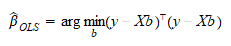that is, it minimizes the sum of squared residuals.

The GLS estimator can be shown to solve the problem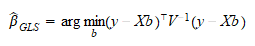which is called generalized least squares problem.

Proof

The first order condition for a maximum iswhose solution isorThe second order derivative iswhich is positive definite (becauseis full-rank andis positive definite). Therefore, the function to be minimized is globally convex and the solution of the first order condition is a global minimum.

The function to be minimized can be written as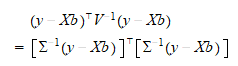It is also a sum of squared residuals, but the original residualsare rescaled bybefore being squared and summed.

## Weighted least squares

When the covariance matrixis diagonal (i.e., the error terms are uncorrelated), the GLS estimator is called weighted least squares estimator (WLS). In this case the function to be minimized becomes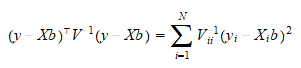whereis the-th entry of,is the-th row of, andis the-th diagonal element of. Thus, we are minimizing a weighted sum of the squared residuals, in which each squared residual is weighted by the reciprocal of its variance. In other words, while estimating, we are giving less weight to the observations for which the linear relationship to be estimated is more noisy, and more weight to those for which it is less noisy.

## Feasible generalized least squares

Note that we need to know the covariance matrixin order to actually compute. In practice, we seldom knowand we replace it with an estimate. The estimator thus obtained, that is,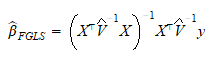is called feasible generalized least squares estimator.

There is no general method for estimating, although the residuals of a fist-step OLS regression are typically used to compute. How the problem is approached depends on the specific application and on additional assumptions that may be made about the process generating the errors of the regression.

Example A typical situation in whichis estimated by running a first-step OLS regression is when the observations are indexed by time. For example, we could assume thatis diagonal and estimate its diagonal elements with an exponential moving average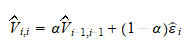where